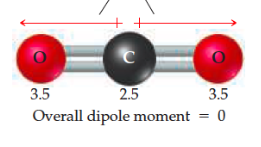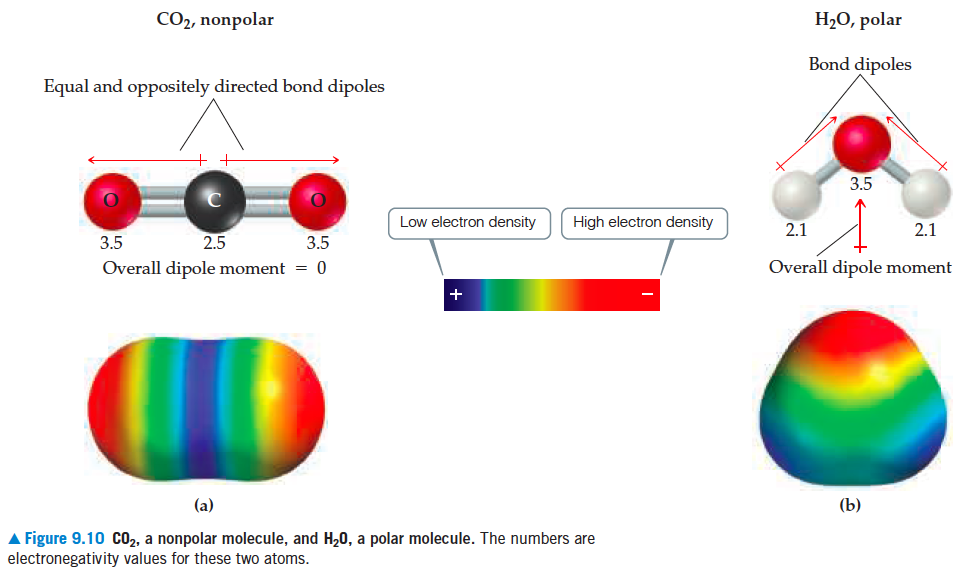# Problem: What is the sum of the two red vectors at the top of the left figure?

###### FREE Expert Solution

We can see that the molecule is linear:• This means that the dipole moments are exactly opposite to each other.
• Also, we can see that they are both O-C bonds, meaning the dipole moment should be the same.

88% (327 ratings)###### Problem Details

What is the sum of the two red vectors at the top of the left figure?